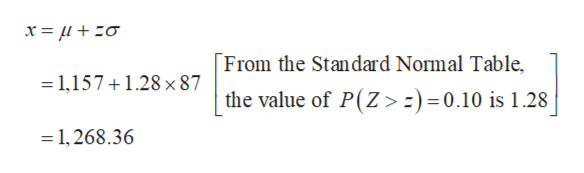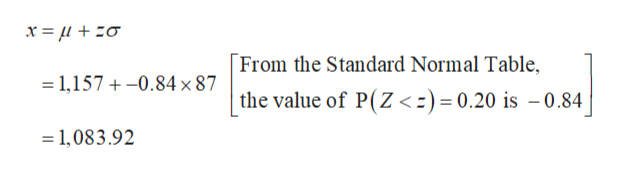How do you solve this problem?Based on the model​N(11571157​,8787​)describing steer​ weights, what are the cutoff values for​a) the highest​ 10% of the​ weights?​b) the lowest​ 20% of the​ weights?​c) the middle​ 40% of the​ weights? ��a) The cutoff weight for the highest​ 10% of the steer weights is _______pounds.

Question

How do you solve this problem?

Based on the model
​N(11571157​,8787​)
describing steer​ weights, what are the cutoff values for
​a) the highest​ 10% of the​ weights?
​b) the lowest​ 20% of the​ weights?
​c) the middle​ 40% of the​ weights?

��a) The cutoff weight for the highest​ 10% of the steer weights is _______
pounds.
Step 1

(a)

The cutoff weight for the highest 10% of the steer weights is obtained as follows:help_outlineImage TranscriptioncloseFrom the Standard Normal Table, 1,1571.28 x 87 the value of P(Z> :) =0.10 is 1.28 1,268.36 fullscreen
Step 2

Thus, the cutoff weight for the highest 10% of the steer weights is 1,268.36 pounds.

(b)

The cutoff weight for the lowest 20% of the steer weights is obtained as follows:help_outlineImage TranscriptioncloseFrom the Standard Normal Table, 1,157-0.84x 87 the value of P(Z<) = 0.20 is -0.84 1,083.92 fullscreen
Step 3

Thus, the cutoff weight for the lowest 20% of the steer weights is 1,083.92 pounds.

...

Want to see the full answer?

See Solution

Want to see this answer and more?

Our solutions are written by experts, many with advanced degrees, and available 24/7

See Solution
Tagged in

Other